# Orthogonal Polynomial

## orthogonal polynomial

[ȯr′thäg·ən·əl päl·ə′nō·mē·əl]
(mathematics)
Orthogonal polynomials are various families of polynomials, which arise as solutions to differential equations related to the hypergeometric equation, and which are mutually orthogonal as functions.
McGraw-Hill Dictionary of Scientific & Technical Terms, 6E, Copyright © 2003 by The McGraw-Hill Companies, Inc.
The following article is from The Great Soviet Encyclopedia (1979). It might be outdated or ideologically biased.

## Orthogonal Polynomial

A set of orthogonal polynomials is a special system of polynomials {pn(x)}, n = 0, 1, 2, …, that are orthogonal with respect to some weight function ρ(x) on an interval [a, b]. A normalized system of orthogonal polynomials is denoted by n(x), and a system of orthogonal polynomials whose leading coefficients are all equal to 1 is denoted by n (x). In boundary value problems of mathematical physics, systems of orthogonal polynomials are often encountered where the weight function ρ(x) satisfies the following differential equation (Pearson’s equation):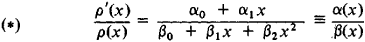A polynomial pn (x) in such a system satisfies the differential equation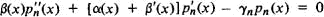where λn = n[α1 + (n + 1) β2].

The most important classical systems of orthogonal polynomials are of this type. Aside from constant multipliers, the polynomials are determined by the choices of a, b, and ρ(x) indicated below.

(1) Jacobi polynomials, {pn(λ,μ)(x)}, correspond to a = –1, b = 1, ρ(x) = (1 – x)λ (1 + x)μ, λ > –1, and μ > –1. Certain values of λ and μ are associated with special cases of Jacobi polynomials. Thus, for λ = μ we have the ultraspherical polynomials, Pn(λ)(x), which are sometimes called Gegen-bauer polynomials. When λ = μ = –½, that is, ρ(x) =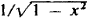we have the Chebyshev polynomials of the first kind, Tn(x); and for λ = μ = ½, that is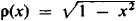, we have the Chebyshev polynomials of the second kind, Un(x). For λ = μ = 0, that is, ρ(x) ≡ 1, we have the Legendre polynomials, Pn(x).

(2) Laguerre polynomials, Ln (x), correspond to a = 0, b = + ∞, and ρ(x) = e–x. For ρ(x) = xae–x >–1), we have the generalized Laguerre polynomials,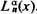.

(3) Hermite polynomials, Hn(x), for a = –, ∞, b = + ∞, and

ρ(x) = ex2

Orthogonal polynomials have many properties in common. The zeroes of the polynomials pn(x) are real and simple and are located within the interval [a, b]. Between any two successive zeroes of pn (x) there lies a zero of pn+1 (x). The polynomial pn (x) is given by Rodrigues’ formula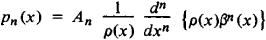where An is a constant and β(x) is of the form given in (*) above. Our systems of orthogonal polynomials are complete. Three successive orthogonal polynomials n(x), n + 1 (x), and n + 2 (x) are related by the recursion formula

n + 2 (x) = (xαn + 2) n + 1)(xλn + 1(x)

where αn + 2 and λn + 1 are given by the coefficients of the polynomials as follows: if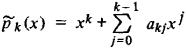then

αn + 2 = αn + 1,nαn + 2, n + 1

λn + 1 = αn + 1, n – 1αn + 2αn + 1, nαn + 2, n

P. L. Chebyshev developed a general theory of orthogonal polynomials. His basic method of studying orthogonal polynomials was to expand the integral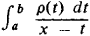in a continued fraction with partial denominators xαn and partial numerators λn – 1. The denominators of the convergents ϕn(x)/pn (x) of this continued fraction form a system of orthogonal polynomials on the interval [a, b] with respect to the weight function ρ(x).

The classical systems of orthogonal polynomials given above can be expressed in terms of the hypergeometric function.

### REFERENCE

Szegö, G. Ortogonal’nye mnogochleny. Moscow, 1962. (Translated from English.)

V. I. BITIUTSKOV

References in periodicals archive ?
The Orthogonal Polynomial Family [{[p.sub.v](x)}.sup.[infinity].sub.v=0].
Neural network with orthogonal polynomial activation function is presented and integrated into the standard LQ control logic.
The second kind of Chebyshev series is orthogonal polynomial with respect to the weighting function [square root of (1- [x.sup.2])]
A 3D displacement function on the surface and thickness direction can be separated, and the thickness direction can be expanded by orthogonal polynomial [{[L.sub.i](z)}.sup.[infinity].sub.0] (Remark 3).
There have been several mathematical methods used to obtain the response of the system with random parameters numerically, for example, Monte Carlo method , stochastic perturbation method [10, 11], and orthogonal polynomial approximation method proposed by Spanos and Ghanem  and Jensen and Iwan  and developed by Li et al.
The polynomial sequence {[p.sub.n](t; [z.sub.1], [z.sub.2], [z.sub.3], q)}n generalizes the orthogonal polynomial system associated with X [4, 13].
In mathematics, the Hermite polynomials are a classical orthogonal polynomial sequence that arise in probability, such as the Edgeworth series; in combinatorics, as an example of an Appell sequence, obeying the umbral calculus; in numerical analysis as Gaussian quadrature; and in physics, where they give rise to the eigenstates of the quantum harmonic oscillator.
In this paper, we used multiple orthogonal polynomial regression design to process the experimental data, considered the interaction of two factors.
Among the 12 mathematical topics are a survey of quadrature rules on the unit circle, the multilineal trigonometric problems of moments, multiple orthogonal polynomial ensembles, greedy energy points with external fields, relative asymptotics for general orthogonal polynomials, a progress report on the fine structure of zeros of orthogonal polynomials, and some open problems.
In [section] 4 the Gegenbauer reconstruction method is first reviewed for one-dimensional problems using both Fourier and orthogonal polynomial expansions.
An orthogonal polynomial model was used to fit the frequency sweep curves for analyzing the elastic viscous moduli of creme fraiche.
Analysis of variance P, K, and the P x K interaction effects were partitioned into orthogonal polynomial contrasts.

Site: Follow: Share:
Open / Close